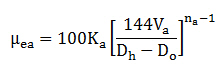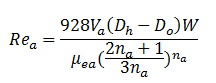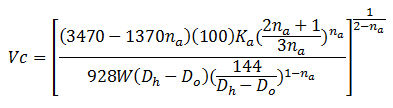## Critical Flow Rate – Drilling Hydraulics

Critical flow rate is the flow rate at the transition point between laminar and turbulent flow. The first step of the critical flow rate determination is to figure out the critical velocity and then substitute it into the annular flow rate.

To get the point at the transition period, the critical Reynold Number for laminar flow must be around 3470 – 1370na. With this relationship, we can determine the critical velocity by rearranging the Reynold Number and Effective Viscosity equation.

The effective viscosity equation for critical velocity is listed below:The Reynolds number equation for critical velocity is listed below:The critical annular velocity equation is listed below: